International
Tables for
Crystallography
Volume C
Mathematical, physical and chemical tables
Edited by E. Prince

International Tables for Crystallography (2006). Vol. C, ch. 1.1, p. 5

Section 1.1.4. The Miller formulae

E. Kocha

aInstitut für Mineralogie, Petrologie und Kristallographie, Universität Marburg, Hans-Meerwein-Strasse, D-35032 Marburg, Germany

1.1.4. The Miller formulae

| top | pdf |

Consider four faces of a crystal that belong to the same zone in consecutive order: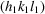,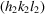,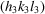, and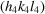. The angles between the ith and the jth face normals are designated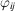. Then the Miller formulae relate the indices of these faces to the angles: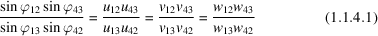with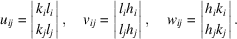If all angles between the face normals and also the indices for three of the faces are known, the indices of the fourth face may be calculated. Equation (1.1.4.1)cannot be used if two of the faces are parallel.

From the definition of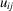,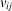, and, it follows that all fractions in (1.1.4.1)are rational: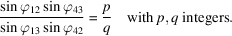Therefore, (1.1.4.1)may be rearranged to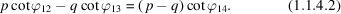This equation allows the determination of one angle if two of the angles and the indices of all four faces are known.